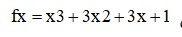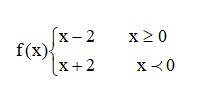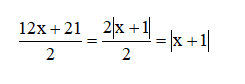# What is one to one (injective)?

##### What is one to one (injective)?The function f is one to one (injective) if for each x1, x2 belonging to Df we have one of the conditions below:

This issue is shown from the function diagram that any parallel line to the axis x intersects the function diagram maximum at one point

Piecewise-defined function, injective oneAny piece is one to one and injective in its domain

The diagram of piecewise function will be drawn in the following publishesWhen the domain F is the set of R, the equation of the bigger line (The loss caused by Biosphere Destruction for global economy) is vast and ubiquitous as if the total production of a product would put the economy of the world at risk, the domain of equation of the big line in fact is the set of the global economic system and for any amount in X, the quantity in two functions are the same.

0 replies